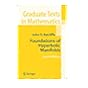Normal view

# Foundations of Hyperbolic Manifolds [electronic resource] / by John G. Ratcliffe.

Material type:TextSeries: Graduate Texts in Mathematics: 149Publisher: New York, NY : Springer New York, 2006Edition: Second EditionDescription: XII, 782 p. online resourceContent type: text Media type: computer Carrier type: online resourceISBN: 9780387473222Additional physical formats: Printed edition:: No titleDDC classification: 516 LOC classification: QA440-699Online resources: Click here to access online
Contents:
Euclidean Geometry -- Spherical Geometry -- Hyperbolic Geometry -- Inversive Geometry -- Isometries of Hyperbolic Space -- Geometry of Discrete Groups -- Classical Discrete Groups -- Geometric Manifolds -- Geometric Surfaces -- Hyperbolic 3-Manifolds -- Hyperbolic n-Manifolds -- Geometrically Finite n-Manifolds -- Geometric Orbifolds.
Summary: This book is an exposition of the theoretical foundations of hyperbolic manifolds. It is intended to be used both as a textbook and as a reference. The book is divided into three parts. The first part is concerned with hyperbolic geometry and discrete groups. The main results are the characterization of hyperbolic reflection groups and Euclidean crystallographic groups. The second part is devoted to the theory of hyperbolic manifolds. The main results are Mostow’s rigidity theorem and the determination of the global geometry of hyperbolic manifolds of finite volume. The third part integrates the first two parts in a development of the theory of hyperbolic orbifolds. The main result is Poincare«s fundamental polyhedron theorem. The exposition if at the level of a second year graduate student with particular emphasis placed on readability and completeness of argument. After reading this book, the reader will have the necessary background to study the current research on hyperbolic manifolds. The second edition is a thorough revision of the first edition that embodies hundreds of changes, corrections, and additions, including over sixty new lemmas, theorems, and corollaries. The new main results are Schl\¬afli’s differential formula and the \$n\$-dimensional Gauss-Bonnet theorem. John G. Ratcliffe is a Professor of Mathematics at Vanderbilt University.
Tags from this library: No tags from this library for this title.Average rating: 0.0 (0 votes)
Item type Current location Collection Call number Status Date due Barcode Item holdseBook e-Library

Electronic Book@IST

EBook Available
Total holds: 0

Euclidean Geometry -- Spherical Geometry -- Hyperbolic Geometry -- Inversive Geometry -- Isometries of Hyperbolic Space -- Geometry of Discrete Groups -- Classical Discrete Groups -- Geometric Manifolds -- Geometric Surfaces -- Hyperbolic 3-Manifolds -- Hyperbolic n-Manifolds -- Geometrically Finite n-Manifolds -- Geometric Orbifolds.

This book is an exposition of the theoretical foundations of hyperbolic manifolds. It is intended to be used both as a textbook and as a reference. The book is divided into three parts. The first part is concerned with hyperbolic geometry and discrete groups. The main results are the characterization of hyperbolic reflection groups and Euclidean crystallographic groups. The second part is devoted to the theory of hyperbolic manifolds. The main results are Mostow’s rigidity theorem and the determination of the global geometry of hyperbolic manifolds of finite volume. The third part integrates the first two parts in a development of the theory of hyperbolic orbifolds. The main result is Poincare«s fundamental polyhedron theorem. The exposition if at the level of a second year graduate student with particular emphasis placed on readability and completeness of argument. After reading this book, the reader will have the necessary background to study the current research on hyperbolic manifolds. The second edition is a thorough revision of the first edition that embodies hundreds of changes, corrections, and additions, including over sixty new lemmas, theorems, and corollaries. The new main results are Schl\¬afli’s differential formula and the \$n\$-dimensional Gauss-Bonnet theorem. John G. Ratcliffe is a Professor of Mathematics at Vanderbilt University.

There are no comments for this item.Foundations of Hyperbolic Manifolds (Graduate Texts in Mathematics). by Ratcliffe, John. ©2006